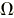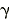# Physics Base & Derived Units

These units are for general physical quantities not specifically related to electricity and magnetism. Below is a much more extensive list derived from the NIST website.

Here is a list of physical constants.

 Name Base Unit Symbol Enthalpy joule H Entropy joule/Kelvin S Heat capacity joule/Kelvin C Internal energy joule U Luminous intensity candela I Quantity of heat joule Q Radiant energy joule W Radiant intensity watt/steradian I Radiant power (flux) watt P Sound intensity watt/meter I Sound energy flux watt W Specific heat capacity joule/(kilogram * Kelvin) c Speed of sound meter/second n Thermal conductivity watt/(meter * Kelvin) l Time Constant s t

## SI Base Units

The SI is founded on seven SI base units for seven base quantities assumed to be mutually independent, as given in Table 1.

## Table 1.  SI base units

 Base Quantity SI Base Unit Name Symbol length meter m mass kilogram kg time second s electric current ampere A thermodynamic temperature kelvin K amount of substance mole mol luminous intensity candela cd

For detailed information on the SI base units, see Definitions of the SI base units and their Historical context.

## SI derived units

Other quantities, called derived quantities, are defined in terms of the seven base quantities via a system of quantity equations. The SI derived units for these derived quantities are obtained from these equations and the seven SI base units. Examples of such SI derived units are given in Table 2, where it should be noted that the symbol 1 for quantities of dimension 1 such as mass fraction is generally omitted.

## Table 2.  Examples of SI derived units

 Name Symbol Derived Quantity SI Derived Unit area square meter m2 volume cubic meter m3 speed, velocity meter per second m/s acceleration meter per second squared m/s2 wave number reciprocal meter m-1 mass density kilogram per cubic meter kg/m3 specific volume cubic meter per kilogram m3/kg current density ampere per square meter A/m2 magnetic field strength ampere per meter A/m amount-of-substance concentration mole per cubic meter mol/m3 luminance candela per square meter cd/m2 mass fraction kilogram per kilogram, which may be      represented by the number 1 kg/kg = 1

For ease of understanding and convenience, 22 SI derived units have been given special names and symbols, as shown in Table 3.

## Table 3.  SI derived units with special names and symbols

 Derived Quantity SI Derived Unit Name Symbol Expressionin terms ofother SI units Expressionin terms ofSI base units plane angle radian (a) rad - m·m-1 = 1 (b) solid angle steradian (a) sr (c) - m2·m-2 = 1 (b) frequency hertz Hz - s-1 force newton N - m·kg·s-2 pressure, stress pascal Pa N/m2 m-1·kg·s-2 energy, work, quantity of heat joule J N·m m2·kg·s-2 power, radiant flux watt W J/s m2·kg·s-3 electric charge, quantity     of electricity coulomb C - s·A electric potential difference,electromotive force volt V W/A m2·kg·s-3·A-1 capacitance farad F C/V m-2·kg-1·s4·A2 electric resistance ohmV/A m2·kg·s-3·A-2 electric conductance siemens S A/V m-2·kg-1·s3·A2 magnetic flux weber Wb V·s m2·kg·s-2·A-1 magnetic flux density tesla T Wb/m2 kg·s-2·A-1 inductance henry H Wb/A m2·kg·s-2·A-2 Celsius temperature degree Celsius °C - K luminous flux lumen lm cd·sr (c) m2·m-2·cd = cd illuminance lux lx lm/m2 m2·m-4·cd = m-2·cd activity (of a radionuclide) becquerel Bq - s-1 absorbed dose, specific energy    (imparted), kerma gray Gy J/kg m2·s-2 dose equivalent (d) sievert Sv J/kg m2·s-2 catalytic activity katal kat s-1·mol (a) The radian and steradian may be used advantageously in expressions for derived units to distinguish between quantities of a different nature but of the same dimension; some examples are given in Table 4. (b) In practice, the symbols rad and sr are used where appropriate, but the derived unit "1" is generally omitted. (c) In photometry, the unit name steradian and the unit symbol sr are usually retained in expressions for derived units. (d) Other quantities expressed in sieverts are ambient dose equivalent, directional dose equivalent, personal dose equivalent, and organ equivalent dose.

For a graphical illustration of how the 22 derived units with special names and symbols given in Table 3 are related to the seven SI base units, see relationships among SI units.

Note on degree Celsius. The derived unit in Table 3 with the special name degree Celsius and special symbol °C deserves comment. Because of the way temperature scales used to be defined, it remains common practice to express a thermodynamic temperature, symbol T, in terms of its difference from the reference temperature T0 = 273.15 K, the ice point. This temperature difference is called a Celsius temperature, symbol t, and is defined by the quantity equation

t= T- T0.

The unit of Celsius temperature is the degree Celsius, symbol °C. The numerical value of a Celsius temperature t expressed in degrees Celsius is given by

t/°C = T/K - 273.15.

It follows from the definition of t that the degree Celsius is equal in magnitude to the kelvin, which in turn implies that the numerical value of a given temperature difference or temperature interval whose value is expressed in the unit degree Celsius (°C) is equal to the numerical value of the same difference or interval when its value is expressed in the unit kelvin (K). Thus, temperature differences or temperature intervals may be expressed in either the degree Celsius or the kelvin using the same numerical value. For example, the Celsius temperature differencet and the thermodynamic temperature differenceT between the melting point of gallium and the triple point of water may be written ast = 29.7546 °C =T = 29.7546 K.

The special names and symbols of the 22 SI derived units with special names and symbols given in Table 3 may themselves be included in the names and symbols of other SI derived units, as shown in Table 4.

## Table 4.  Examples of SI derived units whose names and symbols include SI derived units with special names and symbols

 Derived Quantity SI Derived Unit Name Symbol dynamic viscosity pascal second Pa·s moment of force newton meter N·m surface tension newton per meter N/m angular velocity radian per second rad/s angular acceleration radian per second squared rad/s2 heat flux density, irradiance watt per square meter W/m2 heat capacity, entropy joule per kelvin J/K specific heat capacity, specific entropy joule per kilogram kelvin J/(kg·K) specific energy joule per kilogram J/kg thermal conductivity watt per meter kelvin W/(m·K) energy density joule per cubic meter J/m3 electric field strength volt per meter V/m electric charge density coulomb per cubic meter C/m3 electric flux density coulomb per square meter C/m2 permittivity farad per meter F/m permeability henry per meter H/m molar energy joule per mole J/mol molar entropy, molar heat capacity joule per mole kelvin J/(mol·K) exposure (x andrays) coulomb per kilogram C/kg absorbed dose rate gray per second Gy/s radiant intensity watt per steradian W/sr radiance watt per square meter steradian W/(m2·sr) catalytic (activity) concentration katal per cubic meter kat/m3About RF CafeCopyright: 1996 - 2024Webmaster:    Kirt Blattenberger,    BSEE - KB3UON RF Cafe began life in 1996 as "RF Tools" in an AOL screen name web space totaling 2 MB. Its primary purpose was to provide me with ready access to commonly needed formulas and reference material while performing my work as an RF system and circuit design engineer. The World Wide Web (Internet) was largely an unknown entity at the time and bandwidth was a scarce commodity. Dial-up modems blazed along at 14.4 kbps while typing up your telephone line, and a nice lady's voice announced "You've Got Mail" when a new message arrived... All trademarks, copyrights, patents, and other rights of ownership to images and text used on the RF Cafe website are hereby acknowledged. My Hobby Website:  AirplanesAndRockets.comPlease Support RF Cafe by purchasing my  ridiculously low−priced products, all of which I created. These Are Available for Free